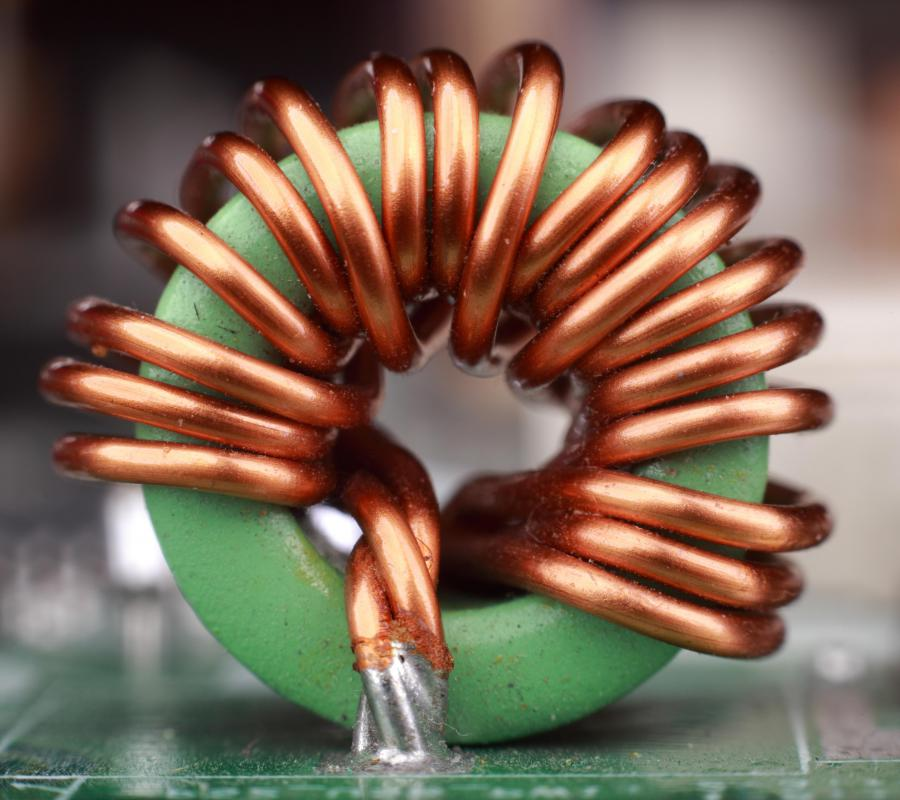# What are Inductors?

Brendan McGuigan
Brendan McGuiganInductors are used in electrical devices such as radio frequency circuits, transformers, motors and generators.

Inductors are electrical devices used in a number of applications, particularly radio frequency circuits. Inductors are closely related to capacitors and can in many ways be considered their opposite. In a capacitor, the energy is determined by the capacitance and the voltage, while in an inductor, the equation is the same, except that inductance replaces capacitance.

Inductors are labeled by their rate of inductance, which is given in the standard unit of henrys (H). An easy one-to-one definition of henrys can be found by taking an inductor of 1 henry and running 1 volt across it, which will result in a current increasing by 1 amp/s. We can present this as an equation of V = L(di/dt), where V is the voltage, L is a measurement of henrys, di is a change in current, and dt is a rate of time.

The most basic inductors are simply tightly wrapped coils of wire. Usually, inductors are wrapped around some sort of core material in order to increase the inductance of the wire. Iron is far and away the most common core material used for inductors, though ferrite is also widely used.

Any conductor will have some amount of inductance, simply because there is a current to be opposed. Inductors are particularly designed to create this inductance, however. When we speak of inductance, we are talking about a magnetic field that is created by changing the current that runs through a medium. By wrapping a wire into a coil around itself, the amount of current necessary to create a magnetic field of a given power is reduced accordingly, and the more times the wire is wrapped, the less current is needed. By using a core material like iron, rather than letting the coil surround air, the magnetic field created by the inductor is held in to the inductor body, increasing the overall inductance.

Inductors are commonly used in radio frequency devices to quiet interference and prevent it from being transmitted. They are used in making transformers, as well. A transformer is, in essence, simply two or more inductors placed together so that their magnetic fields overlap.

## You might also Like## Discuss this Article

Forgot password?
###### Register:
•Inductors are used in electrical devices such as radio frequency circuits, transformers, motors and generators.